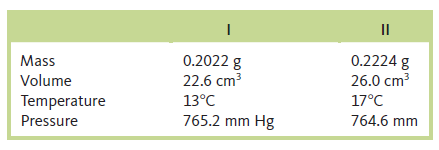# Problem: One of the chemical controversies of the nineteenth century concerned the element beryllium (Be). Berzelius originally claimed that beryllium was a trivalent element (forming Be3+ ions) and that it gave an oxide with the formula Be2O3. This resulted in a calculated atomic mass of 13.5 for beryllium. In formulating his periodic table, Mendeleev proposed that beryllium was divalent (forming Be2+ ions) and that it gave an oxide with the formula BeO. This assumption gives an atomic mass of 9.0. In 1894, A. Combes (Comptes Rendus 1894, p. 1221) reacted beryllium with the anion C5H7O2- and measured the density of the gaseous product. Combes’s data for two different experiments are as follows:If beryllium is a divalent metal, the molecular formula of the product will be Be(C 5H7O2)2; if it is trivalent, the formula will be Be(C5H7O2)3. Show how Combes’s data help to confirm that beryllium is a divalent metal.

⚠️Our tutors found the solution shown to be helpful for the problem you're searching for. We don't have the exact solution yet.

###### Problem Details

One of the chemical controversies of the nineteenth century concerned the element beryllium (Be). Berzelius originally claimed that beryllium was a trivalent element (forming Be3+ ions) and that it gave an oxide with the formula Be2O3. This resulted in a calculated atomic mass of 13.5 for beryllium. In formulating his periodic table, Mendeleev proposed that beryllium was divalent (forming Be2+ ions) and that it gave an oxide with the formula BeO. This assumption gives an atomic mass of 9.0. In 1894, A. Combes (Comptes Rendus 1894, p. 1221) reacted beryllium with the anion C5H7O2- and measured the density of the gaseous product. Combes’s data for two different experiments are as follows:If beryllium is a divalent metal, the molecular formula of the product will be Be(C 5H7O2)2; if it is trivalent, the formula will be Be(C5H7O2)3. Show how Combes’s data help to confirm that beryllium is a divalent metal.